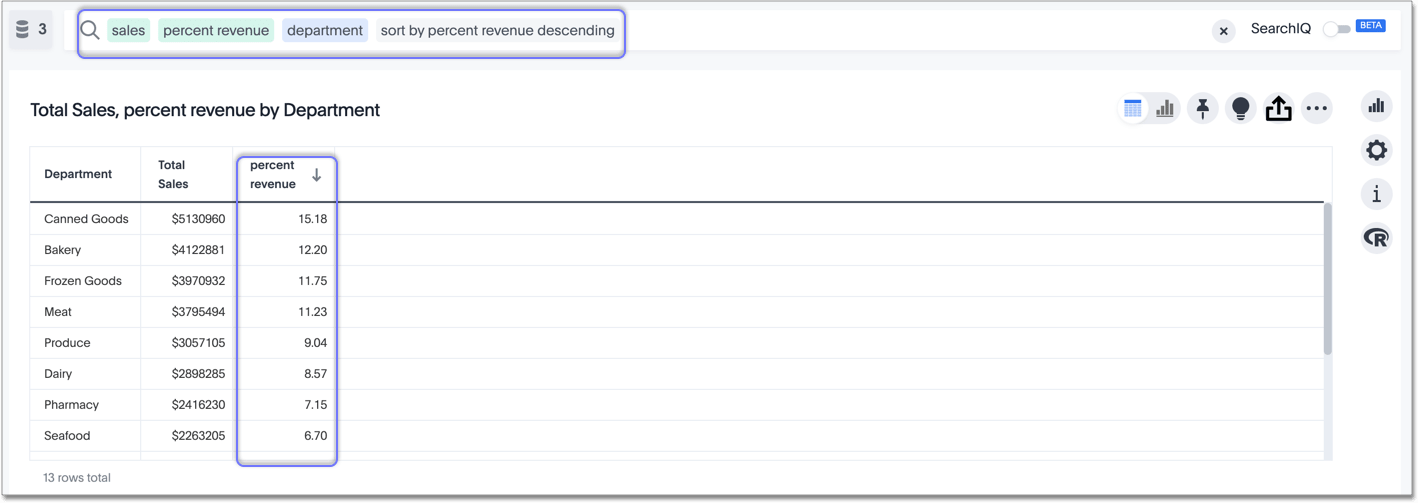# Percent calculations

You can use Simple number calculations to perform useful percent calculations on your data. Simple number functions include addition, subtraction, multiplication, and division.

Calculating percentages is useful when you want to see, for example, the percentage of revenue that each retail department generates (gifts, bakery, canned goods, and so on).

Create a formula called `Percent Revenue` in the Formula Assistant:

`( sum ( revenue ) / group_sum ( revenue ) ) * 100`

Replace `revenue` with your company’s name for their `revenue` column, such as `revenue_dollar_amount`.

Now you can see the percentage of your total revenue that each department contributes, using the percent revenue formula that you just created.